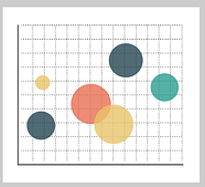# Types of Research Hypotheses

There are seven different types of research hypotheses.Simple Hypothesis
A simple hypothesis predicts the relationship between a single dependent variable and a single independent variable.
Complex Hypothesis
A complex hypothesis predicts the relationship between two or more independent and dependent variables.
Directional Hypothesis
A directional hypothesis specifies the expected direction to be followed to determine the relationship between variables. This kind of hypothesis is derived from theory, and it also implies the researcher’s academic commitment to a particular outcome.
Non-directional Hypothesis
A non-directional hypothesis does not predict the exact direction or nature of the relationship between the two variables. A researcher would utilize this kind of hypothesis if it is not based on theory or when the new research findings contradict the previous research.
Associative and Causal Hypothesis
An associative hypothesis defines the relationship and interdependency between variables. For example, an associative hypothesis predicts that a change in one variable will result in a change of the other variable.A causal hypothesis, on the other hand, proposes that there will be an effect on the dependent variable as a result of a manipulation of the independent variable.
Null Hypothesis
A null hypothesis, denoted by H0, posits a negative statement to support the researcher’s findings that there is no relationship between two variables or that any findings are the result of mere chance.
Alternative Hypothesis
An alternative hypothesis, denoted by H1 or Ha, challenges the null hypothesis and states that there is a relationship between the two variables of the study and that the results are significant to the research topic.